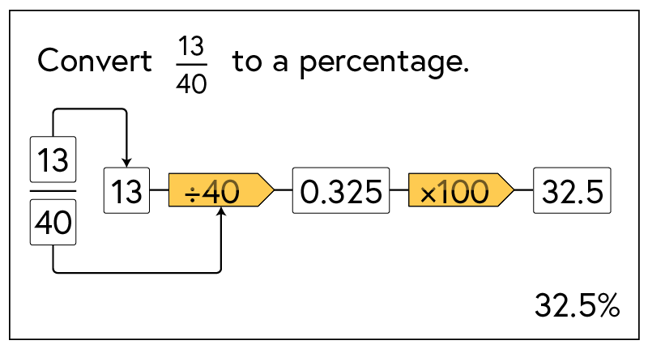## Want to keep learning?

This content is taken from the National STEM Learning Centre's online course, Maths Subject Knowledge: Fractions, Decimals, and Percentages. Join the course to learn more.
2.9

# Converting a fraction to a percentage

When converting from a fraction to a percentage, we aim to find an equivalent fraction where the denominator is 100.

However, it is not always obvious how to scale a fraction so that the denominator is 100. We can therefore apply the knowledge we have about the connections between fractions decimals and percentages.

To convert a fraction to a percentage we need two facts:

• We can express a fraction as a decimal by dividing the numerator by the denominator.
• We can express a decimal as a percentage by multiplying by 100.

The following flow chart shows how $$\frac{13}{40}$$ can be expressed as 0.325 and 32.5%.## Challenge

Express $$\frac{3}{7}$$ as a percentage. Post your answer below.

Highlight any problems or issues your think students may have completing this task.

## Problem worksheet

Now complete questions five and six from this week’s worksheet